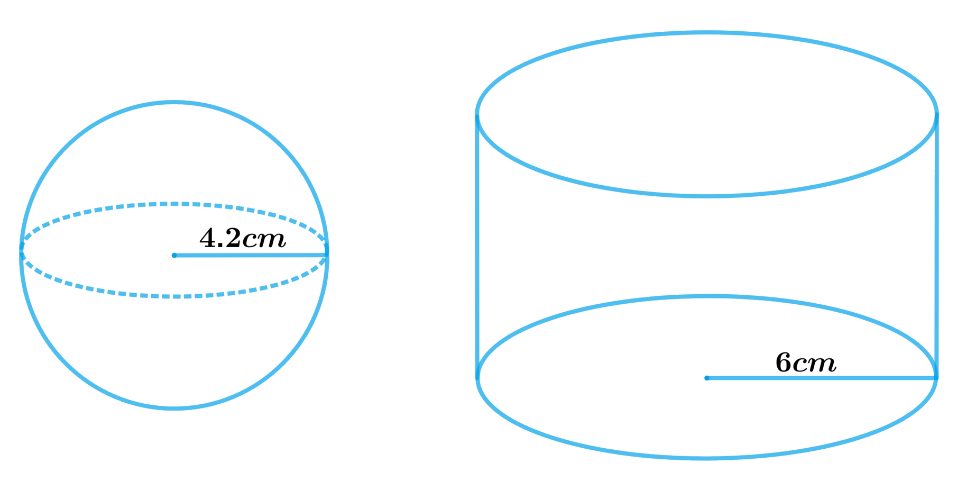# Ex.13.3 Q1 Surface Areas and Volumes Solution - NCERT Maths Class 10

Go back to  'Ex.13.3'

## Question

A metallic sphere of radius $$4.2\rm \,{cm}$$ is melted and recast into the shape of a cylinder of radius $$6\rm \,{cm}$$. Find the height of the cylinder.

Video Solution
Surface Areas And Volumes
Ex 13.3 | Question 1

## Text Solution

What is known?

Radius of the metallic sphere $$4.2 \,\rm{cm}$$ and radius of the cylinder $$6 \,\rm{cm}$$

What is unknown?

The height of the cylinder.

Reasoning:

Draw a figure to visualize the shapes betterSince, a metallic sphere is melted and recast into the shape of a cylinder then their volume must be same.

Volume of the sphere $$=$$ Volume of the cylinder

We will find the volume of the sphere and cylinder by using formulae;

Volume of the sphere\begin{align} = \frac{4}{3}\pi {r^3}\end{align}

where $$r$$ is the radius of the sphere

Volume of the cylinder $$= \pi {r^2}h$$

where $$r$$ and $$h$$ are radius and height of the cylinder respectively

Steps:

Radius of the hemisphere, $${r_1} = 4.2 \rm cm$$

Radius of the cylinder, $${r_2} = 6 \rm cm$$

Let the height of the cylinder be $$h$$.

Volume of sphere $$=$$ Volume of cylinder

\begin{align}\frac{4}{3}\pi r_1^3 &= \,\pi r_2^2h\\\frac{4}{3}r_1^3 &= \,r_2^2h\end{align}

\begin{align}h &= \frac{{4r_1^3}}{{3\,r_2^2}}\\&= \frac{\begin{pmatrix} 4 \times 4.2 \rm cm \times \\ 4.2 \rm cm \times 4.2cm\end{pmatrix} }{{3 \times 6 \rm cm \times 6cm}}\\&= 2.74 \rm cm\end{align}

Hence, the height of the cylinder so formed will be $$2.74\rm{ cm.}$$

Learn from the best math teachers and top your exams

• Live one on one classroom and doubt clearing
• Practice worksheets in and after class for conceptual clarity
• Personalized curriculum to keep up with school